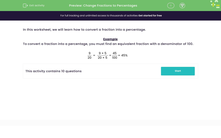# Change Fractions to Percentages

In this worksheet, students will convert fractions into percentages.Key stage:  KS 3

Curriculum topic:   Number

Curriculum subtopic:   Define, Interpret and Compare Percentages

Difficulty level:#### Worksheet Overview

In this activity, we will practise converting a fraction into a percentage.

To convert a fraction into a percentage, you need to find an equivalent fraction with a denominator of 100.

 9 20
=
 9 × 5 20 × 5
=
 45 100
= 45%

The more you practise these, the more familiar you will become with the equivalent values for different denominators. Knowing the factor pairs to make 100 is also really helpful.

For example:

2 x 50 = 100

4 x 25 = 100

5 x 20 = 100

10 x 10 = 100

Let's have a go at another one.

Convert 8/25  to a percentage:

We can get 100 as the denominator if we multiply it by 4.

To create an equivalent fraction, we need to multiply both the numerator and the denominator by the same number:

8/25 x 4 = 32/100, which makes 32%

Are you ready to have a go at some questions?

### What is EdPlace?

We're your National Curriculum aligned online education content provider helping each child succeed in English, maths and science from year 1 to GCSE. With an EdPlace account you’ll be able to track and measure progress, helping each child achieve their best. We build confidence and attainment by personalising each child’s learning at a level that suits them.

Get started